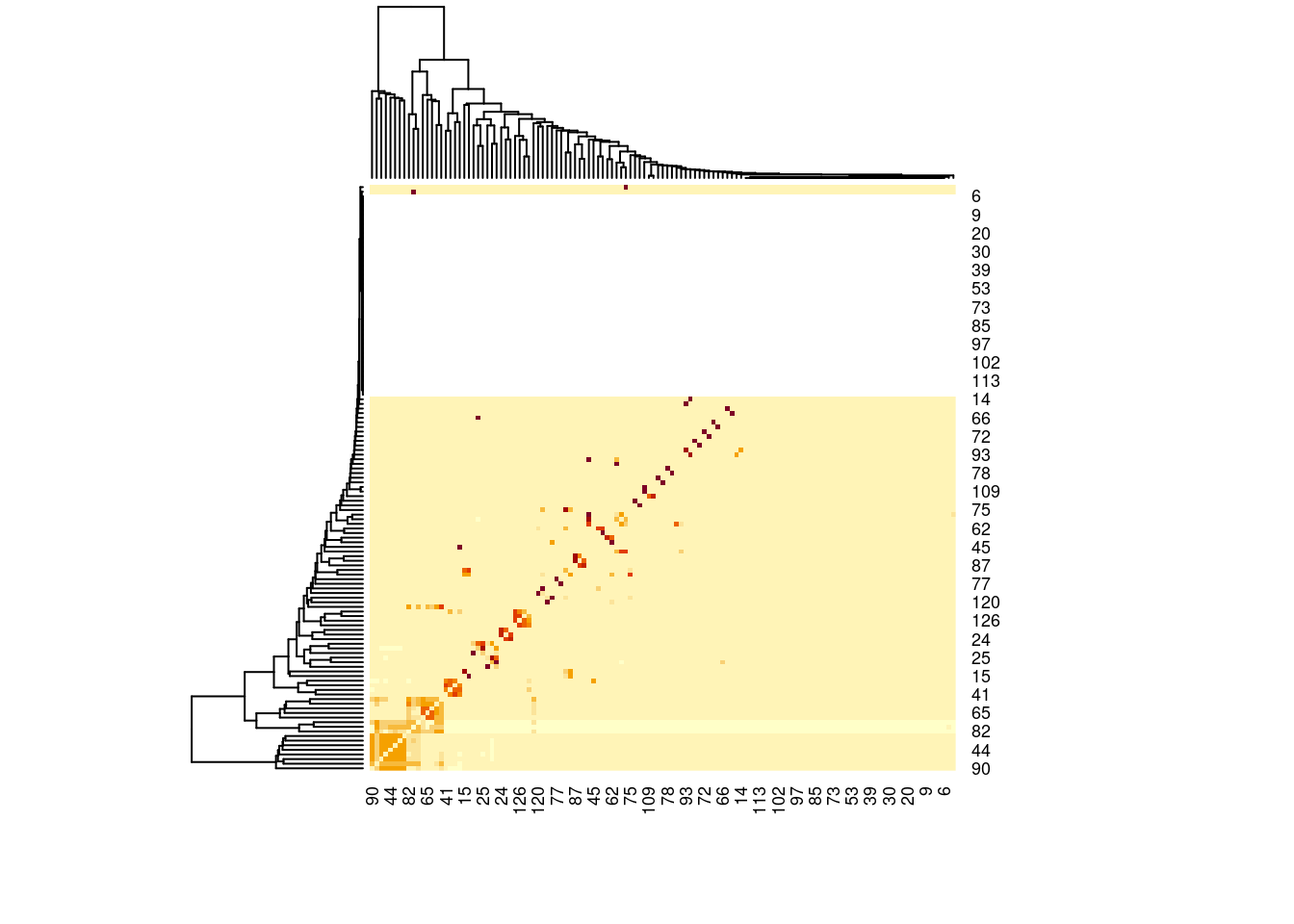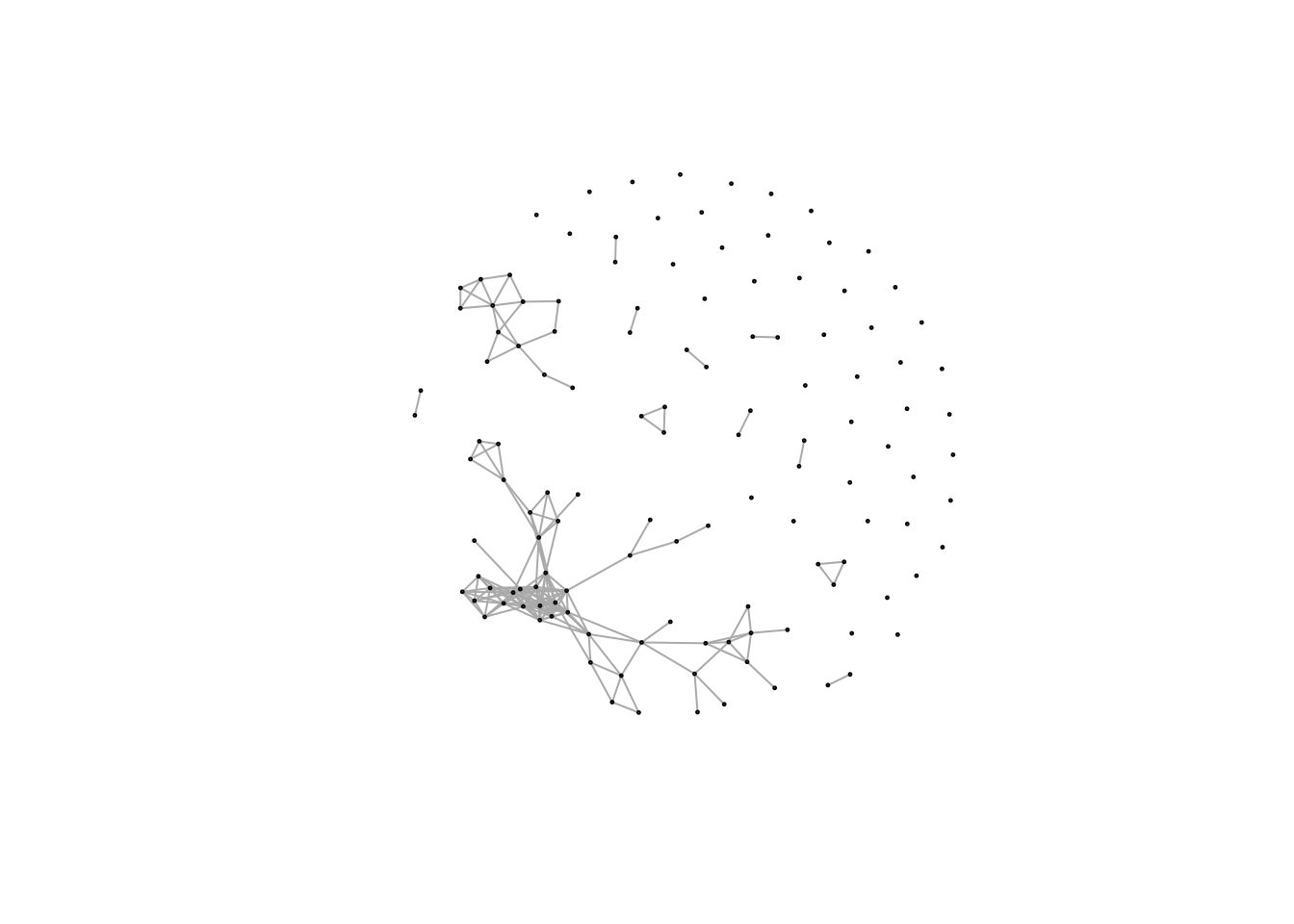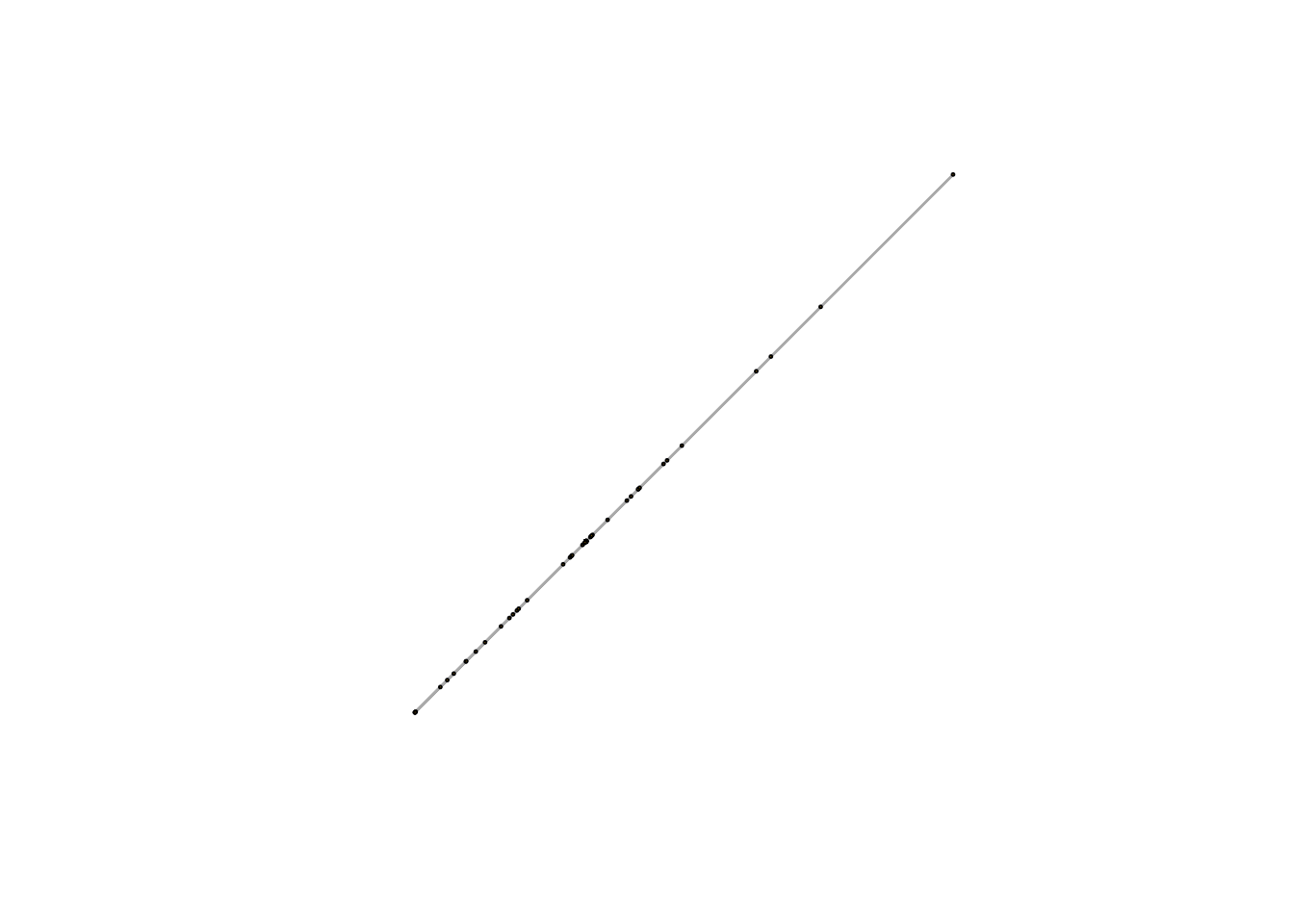Today I am going to take you on a brief tour of the network visiualization capabilities of the igraph package in R (we’ll cover some simple analyses in the next lesson, igraph part II). The igraph package is large and has many functions for manipulating, visualizing, and analyzing your networks. I recommend reading the package documentation, and the many online tutorials available to explore more advanced functionality. For those who prefer ggplot for their plotting needs, the ggnetwork package may be helpful.

Fair warning, igraph’s interface is a little confusing at first. I recommend playing with your network to get a hang of it (“learning through fiddling”“).

## Setup (Making the Network)

First we need a network to visualize. The networks we built in previous lessons are pretty boring visually, so we are going to use a different example today, taken directly from the SpiecEasi documentation. We use their american gut microbiome dataset. See their tutorial for details about this dataset and the functions we use for network construction below.

You should already have installed SpiecEasi and igraph in previous lessons:

``````library(SpiecEasi)
library(igraph)``````
``````##
## Attaching package: 'igraph'``````
``````## The following object is masked from 'package:SpiecEasi':
##
##     make_graph``````
``````## The following objects are masked from 'package:stats':
##
##     decompose, spectrum``````
``````## The following object is masked from 'package:base':
##
##     union``````
``````#Custom colorblind pallette, see: https://stackoverflow.com/questions/57153428/r-plot-color-combinations-that-are-colorblind-accessible
customvermillion<-rgb(213/255,94/255,0/255)
custombluegreen<-rgb(0/255,158/255,115/255)
customblue<-rgb(0/255,114/255,178/255)
customskyblue<-rgb(86/255,180/255,233/255)
customreddishpurple<-rgb(204/255,121/255,167/255) ``````

We build a network using SpiecEasi. I don’t really care what the species in this dataset are, so I’m going to assign them arbitrary names here (either a letter or letter.number combination like “A.1”).

``````## Generate network, see https://github.com/zdk123/SpiecEasi for a nice walkthrough of this process with this example
data(amgut1.filt)
#Make up some Species "Names" since we don't care and the names aren't easily accessible in this matrix (unless you want to insall phyloseq)
colnames(amgut1.filt) <- make.names(rep(LETTERS[1:26],5),unique = T)[1:ncol(amgut1.filt)]
#Build network w/ spieceasi
se.gl.amgut <- spiec.easi(amgut1.filt, method='glasso', lambda.min.ratio=1e-2,
nlambda=20, pulsar.params=list(rep.num=50))``````
``## Applying data transformations...``
``## Selecting model with pulsar using stars...``
``## Fitting final estimate with glasso...``
``## done``

We use the getRefit() function to extract the adjacency matrix from the spieceasi output. This is a square matrix with species on rows and columns and a one if two species are connected (“adjacent”) in the network and a zero otherwise.

``````#Extract adjacency matrix from output  - Explain ADJ MAT vs. EDGE LIST
``````##
##     0     1
## 15773   356``````

But we want a weighted network! No worries, we can extract the weights and make a weighted adjacency matrix as well.

``````#We want weights!
se.cor  <- cov2cor(as.matrix(getOptCov(se.gl.amgut)))

#Let's take a loot at that adjacency matrixIt is very easy to change these adjacency matrices into igraph “graph” objects. What’s a graph? Well a network is a graph (look up “graph theory”, it’s a whole field of mathematics). Consider them synonyms.

``````grph.unweighted <- adj2igraph(adj.mat)

You might also find the graph_from_edgelist() and graph_from_data_frame() functions useful if you have data stored in different formats. If we are worried about self-loops or redundant edges we could also use the simplify() function to remove them (it turns the network into a “simple graph”).

Let’s take a look at our unweighted network:

``plot(grph.unweighted,vertex.size=1,vertex.label=NA)``Great, now our unweighted network:

``````plot(grph,vertex.size=1,
vertex.label=NA)``````Oh no! That looks very bad. It turns out that our graph layout algorithm isn’t behaving well with negative egde weights. Let’s use a different layout function for now (we will get back to this later in the lesson):

``````plot(grph,vertex.size=1,
vertex.label=NA,
edge.width=1,
layout=layout.circle(grph))``````